• +91 9971497814
• info@interviewmaterial.com

# RD Chapter 15- Areas of Parallelograms and Triangles Ex-VSAQS Interview Questions Answers

### Related Subjects

Question 1 :

If ABC and BDE aretwo equilateral triangles such that D is the mid-point of BC,then find ar (ΔABC) : ar (ΔBDE).

Given: (1)ΔABC is equilateral triangle.

(2) ΔBDE is equilateral triangle.

(3) D is the midpoint of BC.

To find: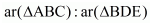PROOF : Let us draw the figure as per theinstruction given in the question.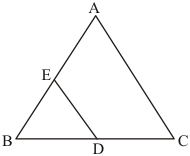We know that area of equilateral triangle =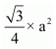, where a is the side of the triangle.

Let us assume that length of BC is a cm.

This means that length of BD is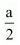cm, Since D is the midpointof BC.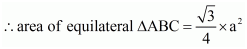------(1)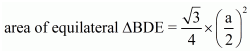------(2)

Now, ar(ÄABC) : ar(ÄBDE) =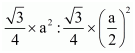(from 1 and 2)

=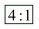Hence we get the result ar(ÄABC) : ar(ÄBDE) =Question 2 : In the given figure, ABCD is a rectangle in which CD = 6 cm, AD = 8 cm. Find the area of parallelogram CDEF.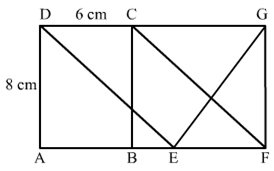Given: (1) ABCD is a rectangle.
(2) CD = 6 cm
To find: Area of rectangle CDEF.
Calculation: We know that,
Area of parallelogram = base × height
The Area of parallelogram and a rectangle on the same base and between the same parallels are equal in area.Here we can see that rectangle ABCD and Parallelogram CDEF are between the same base and same parallels.
Hence,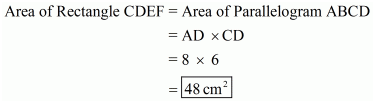Hence we get the result as Area of Rectangle CDEF =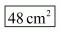Question 3 : In the given figure, find the area of ΔGEF.

Given: (1) ABCD is a rectangle.
(2) CD = 6 cm
To find: Area of ΔGEF.
Calculation: We know that,
Area of Parallelogram = base × height
If a triangle and a parallelogram are on the same base and between the same parallels , the area of the triangle is equal to half of the parallelogram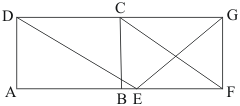Here we can see that Parallelogram ABCD and triangle GEF are between the same base and same parallels.
Hence,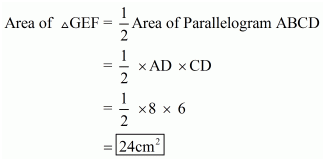Hence we get the result as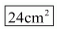Question 4 : In the given figure, ABCD is a rectangle with sides AB = 10 cm and AD = 5 cm. Find the area of ΔEFG.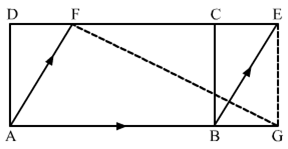Given: (1) ABCD is a rectangle.
(2) AB = 10 cm
To find: Area of ΔEGF.
Calculation: We know that,
Area of Rectangle = base × height
If a triangle and a parallelogram are on the same base and between the same parallels, the area of the triangle is equal to half of the parallelogramHere we can see that Rectangle ABCD and triangle GEF are between the same base and same parallels.
Hence,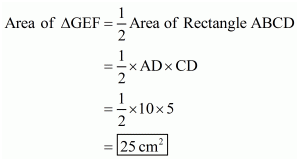Hence we get the result as Area of ÄGEF =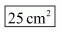Question 5 : PQRS is a rectangle inscribed in a quadrant of a circle of radius 13 cm. A is any point on PQ. If PS = 5 cm, then find ar (ΔRAS)

Given: Here from the given figure we get
(1) PQRS is a rectangle inscribed in a quadrant of a circle with radius 10cm,
(2) PS = 5cm
To find: Area of ΔRAS.
Calculation: In right ΔPSR, (Using Pythagoras Theorem)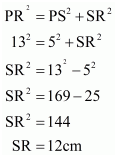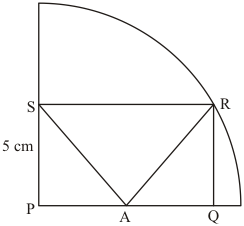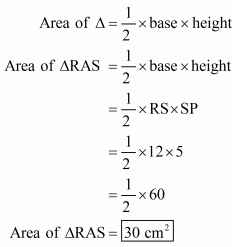Hence we get the Area of ÄRAS =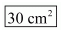Question 6 :

In square ABCDP and Q aremid-point of AB and CD respectively. If AB =8cm and PQ and BD intersect at O,then find area of ΔOPB.

Given: Here from thegiven question we get

(1) ABCD is a square,

(2) P is the midpoint ofAB

(3) Q is the midpoint ofCD

(4) PQ and BD intersectat O.

(5) AB = 8cm

To find : Area of ΔOPB

Calculation: Since P is themidpoint of AB,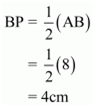BP = 4cm ……(1)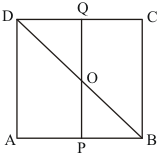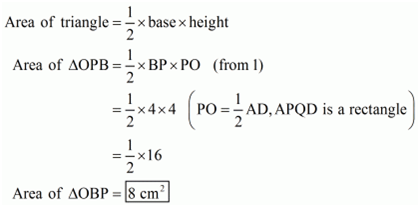Hence we get the Areaof ÄOBP =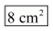Question 7 : ABC is a triangle in which D is the mid-point of BC. E and F are mid-points of DC and AE respectively. IF area of ΔABC is 16 cm2, find the area of ΔDEF.

Given: Here from thegiven question we get

(1) ABC is a triangle

(2) D is the midpoint ofBC

(3) E is the midpoint ofCD

(4) F is the midpoint ofA

Area of ÄABC = 16 cm2

To find : Area of ΔDEF

Calculation: We know that ,

The median divides a triangle in two triangles of equal area.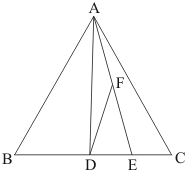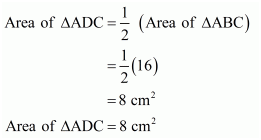For ΔADC , AE is themedian .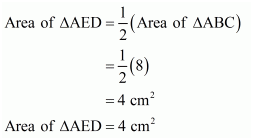Similarly, For ΔAED , DFis the median .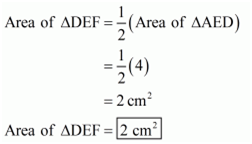Hence we get Areaof ÄDEF =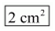Question 8 : PQRS is a trapezium having PS and QR as parallel sides. A is any point on PQ and B is a point on SR such that AB || QR. If area of ΔPBQ is 17cm2, find the area of ΔASR.

Given: Here from the given figure we get
(1) PQRS is a trapezium having PS||QR
(2) A is any point on PQ
(3) B is any point on SR
(4) AB||QR
(5) Area of BPQ = 17 cm2
To find : Area of ΔASR.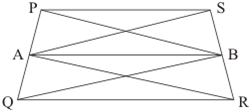Calculation: We know that ‘If a triangle and a parallelogram are on thesame base and the same parallels, the area of the triangle is equal to half thearea of the parallelogram’

Here we can see that:

Area (ΔAPB) = Area(ΔABS) …… (1)

And, Area (ΔAQR) = Area(ΔABR) …… (2)

Therefore,

Area (ΔASR) = Area(ΔABS) + Area (ΔABR)

From equation (1) and(2), we have,

Area (ΔASR) = Area(ΔAPB) + Area (ΔAQR)

Area (ΔASR) = Area (ΔBPQ) = 17 cm2

Hence, the area of thetriangle ΔASR is 17 cm2.

Question 9 : ABCD is a parallelogram. P is the mid-point of AB. BD and CP intersect at Q such that CQ : QP = 3.1. If ar (ΔPBQ) = 10cm2, find the area of parallelogram ABCD.

It is given that CQ : QP= 3: 1 and Area (PBQ) = 10 cm2

Let CQ = x and QP = 3x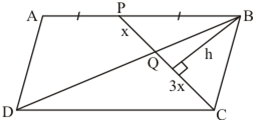We need to find area ofthe parallelogram ABCD.

From the figure,

Area (PBQ) =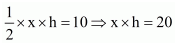And,

Area (BQC) =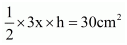Now, let H be theperpendicular distance between AP and CD. Therefore,

Area (PCB) =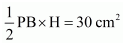…… (1)

Thus the area of theparallelogram ABCD is,

Area (ABCD) = AB × H

Area (ABCD) = 2BP × H

From equation (1), weget

Area (ABCD) = 4 × 30 =120 cm2

Hence, the area of theparallelogram ABCD is 120 cm2.

Question 10 : P is any point on base BC of ΔABC and D is the mid-point of BC. DE is drawn parallel to PA to meet AC at E. If ar (ΔABC) = 12 cm2, then find area of ΔEPC.

Given: Area (ABC) = 12 cm2,D is midpoint of BC and AP is parallel to ED. We need to find area of thetriangle EPC.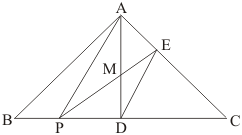Since, AP||ED, and weknow that the area of triangles between the same parallel and on the same baseare equal. So,

Area (APE) = Area (APD)

Area (APM) + Area (AME) = Area (APM) + Area(PMD)

Area (AME) = Area (PMD) …… (1)

Since, median dividetriangles into two equal parts. So,

Area (ADC) =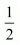Area (ABC) =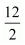= 6 cm2

Area (ADC) = Area (MDCE) + Area (AME)

Area (ADC) = Area(MDCE) + Area (PMD) (from equation (1))

Therefore,

Area (PEC) = 6 cm2.

Todays Deals### RD Chapter 15- Areas of Parallelograms and Triangles Ex-VSAQS Contributorskrishan

Name:
Email:

# Latest News# 9000 interview questions in different categories